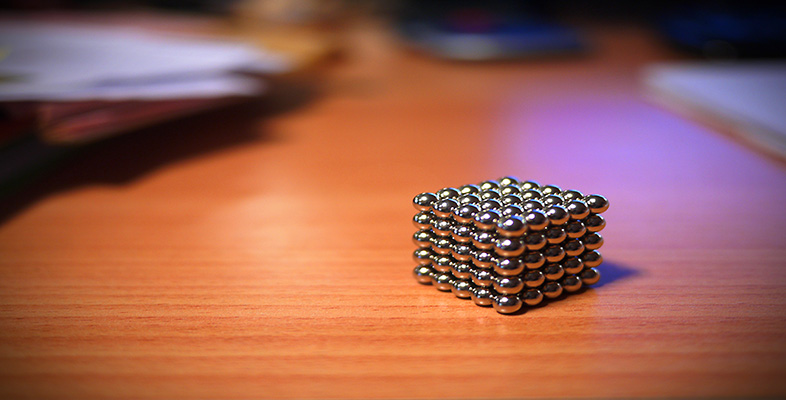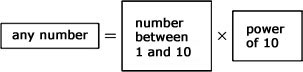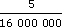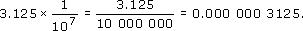Squares, roots and powers

This free course is available to start right now. Review the full course description and key learning outcomes and create an account and enrol if you want a free statement of participation.

Free course

3 Scientific notation

3.1 Expressing numbers in scientific notation

Earlier you looked at place values for numbers, and why they were called powers of ten.

 Place value 10 000 1000 100 10 1 0.1 0.01 0.001 0.0001 0.000 01 Power of ten 104 103 102 101 100 10−1 10−2 10−3 10−4 10−5

Using this notation, very large numbers or small decimal numbers can be expressed in a particularly neat form. This is how scientific and graphics calculators display numbers which will not fit on the screen. This is illustrated by the following examples:

107 = 10 000 000 so 25 000 000 = 2.5 × 107

10−6 = 0.000 001 so 0.000 0025 = 2.5 × 10−6

A number expressed in this form is said to be in scientific notation. Any decimal number can be converted to scientific notation, which isA calculator automatically uses scientific notation when the answer to a calculation is very large or very small. For example, return to our tale of the king and the bags of rice. In week 52 the woman would receive 252 bags. Calculate this on your calculator. You should get 4.503 599 627 × 1015. (This number may be displayed as 4.503 599 627 E 15.) To get a feel for how big this number is, i.e. for how many bags of rice the king would need to provide a year later, look at some other numbers in scientific notation:

Great Britain has an area of 229 850 km2.

In scientific notation, 229 850 = 2.2985 × 100 000 = 2.2985 × 105.

The distance from the Earth to the Sun is 149 600 000 km.

In scientific notation, 149 600 000 = 1.496 × 100 000 000 = 1.496 × 108.

Consider now the bags of rice calculation. 1015 is 1000 000 000 000 000. So the number of bags of rice is 4503 599 627 000 000. This is a very large number of bags of rice! The king would need to give the woman over four thousand million million bags of rice in week 52. No wonder his accountant was concerned.

As mentioned above, scientific notation is also used for very small numbers.

Try findingon your calculator.

You should find that the answer on your calculator is 3.125 × 10−7.

(This number may be displayed as 3.125 E −7.) This time the index is negative. This is the same as:With practice you may not need the intermediate steps. You may be able to just move the decimal point the appropriate number of places (multiplying by 10−3 means moving the decimal point 3 places to the left).

5.2 × 10−3 = 0.0052

MU120_4M4